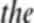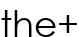# Transformer-based OCR model not learning

Would appreciate any thoughts or references to papers that might be relevant. I’m trying to build a simple OCR model (see example images from the MNIST words dataset) where I encode image features using `vgg` and feed those features into a Transformer. Everything is “working” in the sense that it seems to be going through the training without throwing any errors. But the results so far have been nonsense, although the training error does improve somewhat over the first few epochs. Here are the two main classes. I guess the most important question to address first is whether this architecture should work if done correctly (perhaps, I’m doing something incorrectly).``````class FeatureExtractor(torch.nn.Module):
def __init__(self):
super(FeatureExtractor, self).__init__()
vgg = torchvision.models.vgg16(pretrained=False)
self.cnn = torch.nn.Sequential()
vgg_modules = list(vgg.children())[:-1]
for i in range(len(vgg_modules)):
torch.nn.Flatten(start_dim=2),
torch.nn.Linear(in_features=49, out_features=96)
))
self.pos_encoder = PositionalEncodingTrig(96, 0.1)

def forward(self, x):
x = self.cnn(x)
x = x.reshape(512, -1, 96)
x = self.pos_encoder(x)
return x
``````
``````class OCRModel(torch.nn.Module):
def __init__(self):
super(OCRModel, self).__init__()
self.fe = FeatureExtractor()